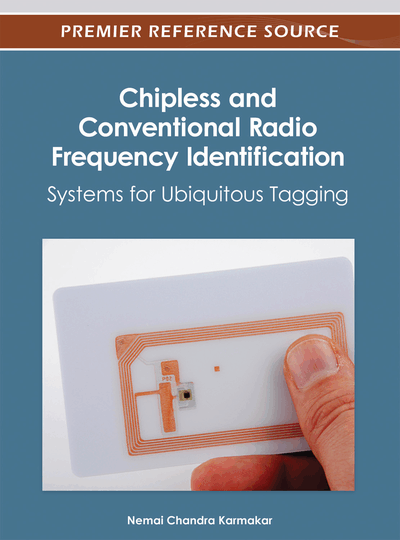# Analysis and Design of Meander Line Dipole Antennas

Zhonghao Hu (Auto-ID Lab, The University of Adelaide, Australia), Peter H. Cole (Auto-ID Lab, The University of Adelaide, Australia), Christophe Fumeaux (The University of Adelaide, Australia) and Yuexian Wang (The University of Adelaide, Australia)
DOI: 10.4018/978-1-4666-1616-5.ch002
Available
\$37.50
No Current Special Offers

## Abstract

A simple analytic formula, found in the literature for calculating the resonant frequency of a meander line dipole antenna (MDA) in free space from its physical parameters is described. The formula is modified to calculate the resonant frequency of an MDA on a dielectric substrate and for use as an RFID tag antenna by taking two factors into account: (i) the effects of dielectric material underneath the MDA, (ii) the special needs of an impedance matching condition in RFID tag antenna design. The parameter of relative effective permittivity for an MDA on a dielectric board, and the method for deriving this parameter, are introduced. Experiments to verify the modified formula are reported. Test results such as input impedance and reading range of an RFID tag antenna design based on an MDA on a dielectric board are provided. Following that, the radiation pattern and efficiency of an MDA either in free space or on a board are investigated.
Chapter Preview
Top

## 1. Introduction

Nowadays the meander line dipole antenna (MDA) is used widely in UHF RFID tag antenna design (Choi & Shin et al., 2006), (Marrocco, 2008) because of its size reduction property and relative high radiation efficiency. The MDA is actually a dipole loaded with meander lines. One example of an MDA loaded with six meander lines is shown in Figure 1. Significant research work has been done on MDAs. Nakano, Tagami, Yoshizawa & Yamauchi (1984) inspired by the appearance of the meander monopole antenna (Rashed & Tai, 1982), firstly proposed the meander line dipole antenna (MDA). Nakano, Tagami, Yoshizawa & Yamauchi (1984) not only proposed the MDA, but also analysed its radiation pattern, input impedance and size reduction ratio relative to the half wavelength dipole, when they are both resonant at the same frequency. The radiation efficiency of the MDA has been studied by Marrocco (2003). According to his research, an approximate current distribution on an MDA loaded with six meander lines is also shown in Figure 1. Since the currents on the adjacent vertical segment of each meander cell are opposite, these currents actually do not contribute to the radiation but bring losses. The radiation resistance is mainly determined by the horizontal segments of the MDA. Moreover, because the large currents occur near the centre of the MDA as shown in Figure 1, one should not place meander lines, especially long meander lines (with large vertical dimension), near the centre. Genetic algorithms (GA) are also used to obtain a gain-optimised MDA within a fixed maximum available area (Marrocco, 2003). However, most analyses of MDAs are based on numerical methods, and to arrive at the optimal MDA design, such numerical computations have to be iterated, with the result that the calculations are extensive. As a result, a simple analytic formula was proposed by Endo, Sunahara, Satoh & Katagi (2000) to calculate the resonant frequency from an MDA’s geometrical parameters.

Figure 1.

A sample of meander line dipole antenna with approximate representation of the instantaneous current distribution. The arrows on the antenna represent the current flow direction and the number of the arrows illustrates the magnitude of the current distribution.

All the literature introduced above including the formula proposed by Endo, Sunahara, Satoh & Katagi (2000) assumes that MDA is working in free space. However, for the purpose of antenna protection, mechanical stability, size reduction (Michishita, Yamada & Nakakura, 2004) and high radiation efficiency (Yamada & Michishita, 2005), large numbers of MDAs are commonly fabricated on dielectric substrates. Although numerical electromagnetic methods such as MOM (Method Of Moments) and FEM (Finite Element Method) can provide us reliable and accurate characteristics of MDAs on dielectric substrates, a simple method is still needed to avoid the burdens of the numerical methods in analysing the MDA.

This chapter aims, by summarising the existing literature, to give a complete analysis of resonant frequency, radiation pattern and radiation efficiency of an MDA and by contributing original thoughts to modify the formula proposed by Endo, Sunahara, Satoh & Katagi (2000) to calculate the resonant frequency of an MDA not only in free space but also above a dielectric board for RFID tag antenna design.

## Complete Chapter List

Search this Book:
Reset